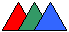Home Math Tim's Skiing Sites#### Median, Bisectrix, Height

The median length is always less than half of the sum of lengths of the sides going from the same vertex. It is more than this half of the sum minus half of the length of the third side.

A triangle angle is less than 90 degrees, is 90 degrees, or is more than 90 degrees if and only if the opposite side is less, equal, or more than twice the median going from the same vertex, respectively.

If BD is a bisectrix in triangle ABC, then BD2 = AB*BC - AD*DC

If AD, BE, and CF are heights of an acute triangle and O is the orthocenter, then AD*AO + BE*BO + CF*CO = (AB2 + BC2 + CA2)/2

In any right triangle, the bisectrix of a right angle divides the angle between the median and the height of the same vertex in two equal angles.

If in a triangle, its median coincides with its height or its height coincides with its bisectrix, then the triangle is isosceles.

If two triangles have two sides equal, respectively, and the medians of the their third sides are equal too, then the triangles are congruent.

If two triangles have one side and two medians of the other two sides equal, respectively, then the triangles are congruent.

If two triangles have three medians equal, respectively, then the triangles are congruent.

If two triangles have one side and both heights of the vertices forming this sisde equal, respectively, then the triangles are congruent.

If AD and CE are two bisectors of an isosceles triangle ABC (AB = BC), then angle BDE is twice angle ADE (the same holds for angles BED and CED).

If point D is inside triangle ABC and the areas of triangles ABD, BCD, and CAD are equal, then D is the centroid of triangel ABC.

#### Incircle, Circumcircle

In any triangle ABC, the distance from the circumcircle center to BC is half of the distance from the orthocenter to A.

The radius of the circumcircle is less than half of the radius of the incircle for any triangle.

In any right triangle, the radius of the incircle is less than half of the hypotenuse height.

The sum of two legs of any right triangle equals the sum of the diameters of the circumcircle and incircle.

If c is the radius of the circumcircle, i is the radius of the incircle of one triangele, and the distance between the centers of these circles is d, then d2 = c2 - 2*c*i.

The radius of the incircle of a triangle of area a and perimeter p equals 2*a/p.

If D is a point lying on the circumcircle of an equilateral triangle ABC, and AD crosses BC, then AD = BD + CD.

The distance from a triangle vertex to the orthocenter is twice the distance from the circumcircle center to the opposite side.

If r is the radius of the incircle and s is the radius of the circumcircle of a right triangle, then the area of this triangle is r*(2*s + r).

#### References

• V.B. Lidskiy, L.V. Ovsyannikov, A.N. Tulaikov, M.I. Shabunin. Problems in Elementary Mathematics. Nauka, Moscow, 1968 (in Russian)
• The Geometry Problem Solver. Research and Education Association, Piscataway, NJ, 2001.
• Moscow Center for Continuing Mathematical Education
Back to Tim's Triangular Page         Min/Max Problems Related To Triangles

When triangle study is over, it is time for a change. Why not to try puzzles now?